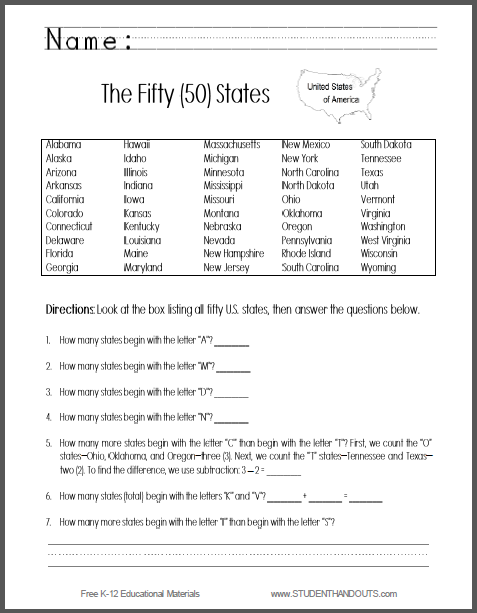Fifty States Math and Geography Worksheet | Student Handouts
 Share the learning joy!

Fifty States - Math and Geography Worksheet
Free Printable Worksheet - Scroll Down to Print (PDF) - Geography - Social Studies - USAHere's a fun worksheet that combines geography and mathematics. Students are presented with a list of the fifty states. Students begin by counting how many states begin with certain letters. Then, students are led to compare states using word problems.

How many more states begin with the letter "O" than begin with the letter "T"? First, we count the "O" states--Ohio, Oklahoma, and Oregon--three (3). Next, we count the "T" states--Tennessee and Texas--two (2). To find the difference, we use subtraction: 3 - 2 = __________